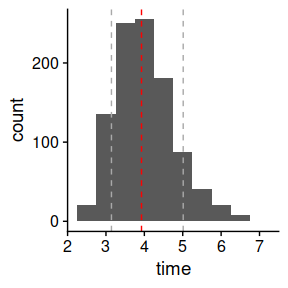Introduction

The growth of populations is of great interest in a variety of scientific fields. However, mechanisms that describe growth are in most cases highly complex and not entirely understood. Hence, population growth is usually analyzed using mathematical models at the population level. Although this approach simplifies the analysis, it still requires the application of advanced mathematical techniques that may limit their application by scientists without a strong background in numerical methods, mathematical modeling or statistical inference.

The biogrowth package includes several functions for modeling population growth. Although the modeling approach is based on predictive microbiology (Perez-Rodriguez and Valero, 2012), the models implemented in the package have also been applied in other fields. The package includes functions for:

• Prediction of population growth under static or dynamic conditions.
• Prediction of population growth under static or dynamic conditions including parameter uncertainty.
• Model fitting of primary growth models to data gathered under static conditions.
• Model fitting of secondary (cardinal) models to growth rates gathered under static conditions.
• One-step model fitting of growth models to data gathered under dynamic conditions.
• Functions to calculate the time required for the population to reach a given size.
• Possibility to fix any model parameter prior to model fitting.

The user can choose among a variety of models for the calculations (described below).

Once installed, the functions included in biogrowth can be loaded with

library(biogrowth)

This command also loads the example datasets that are included in the package.

In this document, we will present the functions included in biogrowth. In order to ease data manipulation, we will be using the tidyverse package.

library(tidyverse)

Several functions in the package make use of stochastic algorithms. For reproducibility, we will set the seed of R’s pseudo-random number generator (PRNG) to some arbitrary value

set.seed(1241)

According to the conventions in predictive microbiology, in this document we will use $$log$$ to refer to the decimal logarithm and $$ln$$ for the natural logarithm.

Mathematical background

Population growth in predictive microbiology

In predictive microbiology mathematical models are usually divided in primary and secondary models (Perez-Rodriguez and Valero, 2012). Primary models describe the variation of the population size against time. These models are largely empirical, so they have model parameters that depend on the environmental conditions. A typical example is the relationship between the specific growth rate of microorganisms and the storage temperature. Predictive microbiology uses so-called, secondary models to describe the relationship between the parameters of the primary model and the environmental conditions.

Primary growth models included in biogrowth

Modified Gompertz model under static conditions

Zwietering et al. (1990) proposed a reparameterization of the Gompertz model with more meaningful parameters parameters. This model predicts the population size $$N(t)$$ for storage time $$t$$ as a sigmoid using the following algebraic equation

$\log_{10} N(t) = \log_{10} N_0 + C \left( \exp \left( -\exp \left( 2.71 \frac{\mu}{C}(\lambda-t)+1 \right) \right) \right)$

where $$N_0$$ is the initial population size, $$\mu$$ is the maximum growth rate, $$\lambda$$ is the duration of the lag phase and $$C$$ is the difference between the initial population size and the maximum population size.

Logistic growth model

The logistic growth model can be parameterized by the following equation (Zwietering et al. 1990)

$\log_{10} N(t) = \log_{10} N_0 + \frac{C}{1 + \exp{ \left(\frac{4 \mu}{C}(\lambda - t)+2 \right) } }$

where $$N_0$$ is the initial population size, $$\mu$$ is the maximum growth rate, $$\lambda$$ is the duration of the lag phase and $$C$$ is the difference between the initial population size and the maximum population size.

Richards growth model

The Richards growth model can be parameterized by the following equation (Zwietering et al. 1990)

$\log_{10} N(t) = \log_{10} N_0 + C \left[1+\nu \cdot \exp{ \left(1 + \nu + \frac{\mu}{A}(1+\nu)^{1+1/\nu} (\lambda - t) \right)} \right]^{-1/\nu}$

where $$N_0$$ is the initial population size, $$\mu$$ is the maximum specific growth rate, $$\lambda$$ is the duration of the lag phase and $$C$$ is the difference between the initial population size and the maximum population size, and $$\nu$$ defines the sharpness of the transition between growth phases.

Baranyi model under dynamic conditions

Baranyi and Roberts (1994) proposed a system of two differential equations to describe microbial growth:

$\frac{dN}{dt} = \frac{Q}{1+Q}\mu'\left(1 - \frac{N}{N_{max}} \right)N$

$\frac{dQ}{dt}=\mu' \space Q$

Note that the maximum specific growth rate is written as $$\mu'$$. The reason for this is that biogrowth makes calculations in log10 scale for the population size. Therefore, for consistency with the equations for static conditions, we use a different notation in these equations. Both parameters are related by the identity $$\mu' = \mu \cdot \log(10)$$.

In the Baranyi model, the deviations with respect to exponential growth are justified based on two hypotheses. It introduces the variable $$Q(t)$$, which represents a theoretical substance that must be produced before the microorganism can enter the exponential growth phase. Hence, its initial value ($$Q_0$$) defines the lag phase duration (under static conditions) as $$\lambda = \frac{\log (1+1/Q_{0})}{\mu}$$. On the other hand, the stationary growth phase is defined by the logistic term $$(1-N/N_{max})$$, which reduces the growth rate as the microorganisms reach the maximum count.

Note that the original paper by Baranyi and Roberts included an exponent, $$m$$, in the term defining the stationary growth phase. However, that term is usually set to 1 by convention in predictive microbiology and, consequently, has been omitted from biogrowth. Also, in its original paper the specific growth rate of $$Q(t)$$ was defined by a different parameter ($$\nu$$). However, because this variable does not correspond to any known substance, it is a convention in the field to set $$\nu = \mu$$.

Baranyi model under static conditions

Oksuz and Buzrul (2020) calculated the solution of the Baranyi model for static conditions given by the following equation

$\log_{10} N = \log_{10} N_{max} + \log_{10}{ \frac{1 + \exp{ \left( \ln (10) \mu (t-\lambda) \right)} - \exp{- \ln (10) \mu \lambda}} {\exp \left( \ln (10) \mu (t-\lambda) \right)- \exp{ \left( - \ln (10) \mu \lambda \right) + 10^{\log_{10} N_{max} - \log_{10} N_0}} } }$

where $$N_0$$ is the initial population size, $$\mu$$ is the maximum specific growth rate and $$N_{max}$$ is the maximum growth rate, and $$\lambda$$ is the lag phase.

Trilinear model under static conditions

Buchanan et al. (1997) proposed a trilinear model as a more simple approach to describe the growth of microbial populations. This model is defined by the piece-wise equation.

The lag phase is defined considering that, as long as $$t < \lambda$$, there is no growth (i.e. $$N = N_0$$).

$\log_{10} N(t) = \log_{10} N_0; t \leq \lambda$ The exponential phase is described considering that during this phase, the specific growth rate is constant, with slope $$\mu_{max}$$.

$\log_{10} N(t) = \log_{10} N_0 + \mu(t-\lambda); t\in(\lambda,t_{max})$

Finally, the stationary phase is modeled considering that once $$N$$ reaches $$N_{max}$$, it remains constant.

$\log_{10} N(t) = \log_{10} N_{max}; t \geq t_{max}$

where $$t_{max}$$, defined is the time required for the population size to reach $$N_{max}$$ ($t_{max} = ( {10} N{max} - _{10} N_0 )/+$).

Gathering primary models metadata directly from biogrowth

The biogrowth package includes the primary_model_data() function, which provides information about the primary models included in the package. It takes just one argument (model_name). By default, this argument is NULL, and the function returns the identifiers of the available models.

primary_model_data()
#>  "modGompertz" "Baranyi"     "Trilinear"   "Logistic"    "Richards"

If a model identifier is passed, it returns a list with mode meta-information.

meta_info <- primary_model_data("Trilinear")

It includes the full reference

meta_info$ref #>  "Buchanan, R. L., Whiting, R. C., and Damert, W. C. (1997). When is simple good enough: A comparison of the Gompertz, Baranyi, and three-phase linear models for fitting bacterial growth curves. Food Microbiology, 14(4), 313-326. https://doi.org/10.1006/fmic.1997.0125" or the identifiers of the model parameters meta_info$pars
#>  "logN0"   "logNmax" "mu"      "lambda"

Secondary growth models included in biogrowth

Although other approaches for secondary modeling exist in the literature, the biogrowth package uses the gamma approach proposed by Zwietering et al. (1992). This approach assumes that the effect of each environmental factor on the growth rate is multiplicative and independent. Hence, the specific growth rate ($$\mu$$) observed under a combination of environmental conditions ($$X_1$$, $$X_2$$,…,$$X_n$$) is the result of multiplying the value of $$\mu$$ observed under optimal conditions for growth ($$\mu_{opt}$$) times one gamma factor for each environmental factor ($$\gamma(X_i)$$). Because gamma factors represent growth rates under sub-optimal conditions, they must take values between 0 and 1.

Note that this approach does not consider interactions between the different terms. The reason for this is that, in the opinion of the authors, their implementation is still a research topic, without any model being broadly accepted. Therefore, the inclusion of interactions between terms is not in line with the philosophy of biogrowth, which aims at easing the application of broadly accepted modeling approaches.

$\mu = \mu_{opt} \cdot \gamma(X_1) \cdot \gamma(X_2) \cdot ... \cdot \gamma(X_n)$

Gamma factors by Zwietering

Zwietering et al. (1992) defined several functions for different environmental factors (temperature, pH and water activity). These functions can be generalized as

$\gamma(X) = \left( \frac{X-X_{min}}{X_{opt}-X_{min}} \right)^n$

where $$X_min$$ is the minimum value of $$X$$ enabling growth, $$X_{opt}$$ is the optimum value of $$X$$ for growth and $$n$$ is the order of the model. The latter parameter usually takes the values 1, 2 or 3, depending on the environmental factor, and the type of population studied.

Cardinal Parameter Model (CPM)

Rosso et al. (1995) defined the following equation for gamma factors

$\gamma(X) = \frac{(X-X_{max})(X-X_{min})^n} {(X_{opt}-X_{min})^{n-1}( (X_{opt}-X_{min})(X-X_{opt}) - (X_{opt}-X_{max}) \cdot ((n-1)X_{opt} + X_{min}-n \cdot X) )}$ with $$\gamma(X) = 0$$ for $$X \notin [X_{min}, X_{max}]$$. In this equation, $$X_{min}$$, $$X_{opt}$$ and $$X_{max}$$ are the minimum, maximum and optimum values of $$X$$ for microbial growth (usually called cardinal parameters). The coefficient $$n$$ is the shape parameter of the cardinal model (usually being fixed to 1, 2 or 3, depending on the case studied).

The full Ratkowsky model (Ratkowsky et al., 1983) is an extension of the square-root model by Ratkowsky (Ratkowsky et al., 1982) that accounts for the decline of the growth rate for temperatures higher than the optimal one. It is described by the following equation

$\sqrt{\mu} = b \left( X-X_{min} \right) \left(1-e^{c(X-X_{max})} \right)$ The application of the gamma concept requires that every gamma function takes values between 0 and 1, whereas the full Ratkowsky model takes values between 0 and $$\sqrt{\mu_{opt}}$$. This can be adapted by defining a gamma function such as

$\gamma_{Ratkowsky}= \left( \frac{\sqrt{\mu}}{ \sqrt{\mu_{opt}} } \right)^2$

For that, we need to know the value of $$\sqrt{\mu_{opt}}$$, which is not included as a parameter in the full Ratkowsky model. By setting the first derivative with respect to $$X$$ to 0 and solving, we get

$X_{opt} = \frac{ W_n \left( e^{-cX_{min} +cX_{max} + 1} \right) + cX_{min} - 1 } {c}$

where $$W_n$$ is the Lambert-W function. Then, the maximum growth rate under optimal conditions is given by

$\sqrt{\mu_{opt}} = b \left( X_{opt}-X_{min} \right) \left(1-e^{c(X_{opt}-X_{max})} \right)$

and the gamma function for the Ratkowsky model can be calculated as

$\gamma_{Ratkowsky}= \left( \frac{\sqrt{\mu}}{ \sqrt{\mu_{opt}} } \right)^2 = \left( \frac{ b \left( X-X_{min} \right) \left(1-e^{c(X-X_{max})} \right)} { b \left( X_{opt}-X_{min} \right) \left(1-e^{c(X_{opt}-X_{max})} \right)} \right)^2 = \left( \frac{ \left( X-X_{min} \right) \left(1-e^{c(X-X_{max})} \right)} {\left( X_{opt}-X_{min} \right) \left(1-e^{c(X_{opt}-X_{max})} \right)} \right)^2$

Note that parameter $$b$$ banishes after doing the division, so this model has 3 model parameters: $$c$$, $$X_{min}$$ and $$X_{max}$$.

Gathering meta data about secondary models directly from biogrowth

The biogrowth package includes the secondary_model_data() function, which provides information about the secondary models it implements. It takes just one argument (model_name). By default, this argument is NULL, and the function returns the identifiers of the available models.

secondary_model_data()
#>  "CPM"           "Zwietering"    "fullRatkowsky"

If, instead, one passes any valid model identifier, the function returns its meta-information.

meta_info <- secondary_model_data("CPM")

This includes the full reference of the model

meta_info$ref #>  "Rosso, L., Lobry, J. R., Bajard, S., and Flandrois, J. P. (1995). Convenient Model To Describe the Combined Effects of Temperature and pH on Microbial Growth. Applied and Environmental Microbiology, 61(2), 610-616." or the identifiers of its model parameters meta_info$pars
#>  "xmin" "xopt" "xmax" "n"

Unit system

The functions in the biogrowth package make the calculations in log10 scale, as this is the common convention in predictive microbiology. This includes also the growth rate, which is defined within the package as the slope of the growth curve during the exponential phase when the plotted in log10 scale versus the elapsed time. Hence, the growth rate used in biogrowth ($$\mu$$), is related to the specific growth rate ($$\mu'$$) by the identity $$\mu = \mu'/\ln(10)$$.

The following table summarizes the unit system used in biogrowth for each quantity. It also describes the identifier used by the functions for each variable/model parameter.

Quantity Identifier Description Unit
$$\log_{10} N$$ logN Decimal logarithm of the population size. log10(units)
$$\log_{10} N_0$$ logN0 Decimal logarithm of the initial population size. log10(units)
$$N$$ N Population size. (units)
$$N_0$$ N0 Initial population size. (units)
$$t$$ t Elapsed time. [time]
$$C$$ C $$\log_{10} N_{max} - \log_{10} N_0$$. log10(units)
$$\mu$$ mu Especific growth rate. log10(units)/[time]
$$\lambda$$ lambda Duration of the lag phase. [time]
$$\nu$$ nu Sharpness of the transition between growth phases in the Richards model. (-)
$$Q$$ Q Variable of the Baranyi model describing the lag phase (units)
$$Q_0$$ Q0 Initial value of $$Q$$. (units)
$$X_{min}$$ xmin Miminum value of $$X$$ enabling growth. [X]
$$X_{opt}$$ xopt Optimum value of $$X$$ for growth. [X]
$$X_{max}$$ xmax Maximum value of $$X$$ enabling growth. [X]
$$n$$ n Order of the secondary model. (-)
$$\mu_{opt}$$ mu_opt Value of $$\mu$$ under optimal environmental conditions for growth. lot10(units)/[time]

The package allows any unit to describe [time], although the user must be consistent in parameter input (e.g. always use hours). Then, predictions and fitted parameters are returned in the same unit system. Regarding population size, the term (units) refers to the unit used to measure the population size. For instance, in predictive microbiology it would be colony forming units (CFU). It can also be a relative magnitude (e.g. CFU/g).

Numerical methods

The biogrowth package uses FME for model fitting and deSolve for estimating population growth under dynamic conditions. Namely, the differential equations required for dynamic calculations are solved using the ode() function from deSolve, which implements several numerical methods. Model fitting is done using the modFit() function from FME for linear regression and the modMCMC() function from the same package for MCMC fitting. The functions in biogrowth use the default settings of these functions, although this can changed using the ... argument included in every relevant function.

Datasets included in the package

The biogrowth package includes several datasets to aid in the understanding of its functions. They can be loaded with a call to the function data() passing the name of the dataset as an argument.

The dataset example_cardinal includes an example of the type of data used for estimating cardinal model parameters. It has three columns: temperature, pH and mu. The two first represent the storage conditions during several static growth experiments, whereas the latter is the specific growth rate observed in those experiments. This dataset is intended to be used for fit_secondary_growth().

data("example_cardinal")
#>   temperature pH           mu
#> 1    0.000000  5 9.768505e-04
#> 2    5.714286  5 2.624919e-03
#> 3   11.428571  5 0.000000e+00
#> 4   17.142857  5 1.530706e-04
#> 5   22.857143  5 2.301817e-05
#> 6   28.571429  5 3.895598e-04

The datasets example_dynamic_growth and example_env_conditions describe a dynamic growth experiment, which can be used for the fit_dynamic_growth() function. The dataset example_env_conditions describes the experimental design; i.e. how the environmental factors vary during the experiment. It has three columns: time (the elapsed time), temperature (the storage temperature) and aw (the water activity).

data("example_env_conditions")
#> # A tibble: 3 x 3
#>    time temperature    aw
#>   <dbl>       <dbl> <dbl>
#> 1     0          20  0.99
#> 2     5          30  0.95
#> 3    15          35  0.9

The dataset example_dynamic_growth illustrates the population size observed during the experiment described by example_env_conditions. It has two columns: time (the elapsed time) and logN (the decimal logarithm of the observed population size).

data("example_dynamic_growth")
#> # A tibble: 6 x 2
#>    time     logN
#>   <dbl>    <dbl>
#> 1 0      0.0670
#> 2 0.517 -0.00463
#> 3 1.03  -0.0980
#> 4 1.55  -0.0986
#> 5 2.07   0.111
#> 6 2.59  -0.0465

The dataset growth_salmonella contains the growth of Salmonella spp. in broth. It has been retrived from ComBase (ID: B092_10). It has two columns: time (elapsed time) and logN (the decimal logarithm of the observed population size).

data("growth_salmonella")
#> # A tibble: 6 x 2
#>    time  logN
#>   <dbl> <dbl>
#> 1  0     3.36
#> 2  1.95  3.4
#> 3  2.78  3.44
#> 4  3.78  3.31
#> 5  4.8   3.39
#> 6  5.7   3.65

The dataset multiple_experiments simulates several growth experiments performed for the same microorganism under dynamic conditions that vary between experiments. It is a nested list with two elements, each describing a single experiment.

data("multiple_experiments")

Each experiment is described using a list with two elements: data and conditions. The former is a tibble with two columns: time (the elapsed time) and logN the observed population size.

head(multiple_experiments[]$data) #> # A tibble: 6 x 2 #> time logN #> <dbl> <dbl> #> 1 0 -0.208 #> 2 1.67 -0.0363 #> 3 3.33 -0.298 #> 4 5 0.350 #> 5 6.67 0.143 #> 6 8.33 -0.404 Then, conditions is also a tibble with one column named time (elapsed time), one called temperature (the storage temperature) and another one called pH (the pH of the media). print(multiple_experiments[]$conditions)
#> # A tibble: 3 x 3
#>    time temperature    pH
#>   <dbl>       <dbl> <dbl>
#> 1     0          20   6.5
#> 2    15          30   7
#> 3    40          40   6.5

The second experiment is described using the same structure.

head(multiple_experiments[]$data) #> # A tibble: 6 x 2 #> time logN #> <dbl> <dbl> #> 1 0 0.533 #> 2 1.67 -0.336 #> 3 3.33 0.0650 #> 4 5 -0.121 #> 5 6.67 0.165 #> 6 8.33 -0.0198 print(multiple_experiments[]$conditions)
#> # A tibble: 3 x 3
#>    time temperature    pH
#>   <dbl>       <dbl> <dbl>
#> 1     0          25     7
#> 2    15          30     6
#> 3    40          25     5

The dataset arabian_tractors includes the number of agricultural tractors in the Arab World according to the World Bank. It is included to show the applicability of fit_isothermal_growth for data from other fields.

data("arabian_tractors")
#> # A tibble: 6 x 2
#>    year tractors
#>   <dbl>    <dbl>
#> 1  1961    73480
#> 2  1962    76900
#> 3  1963    81263
#> 4  1964    86067
#> 5  1965    91117
#> 6  1966    97645

Deterministic modelling

Growth prediction under static conditions

The biogrowth package includes the function predict_isothermal_growth() to make predictions under static conditions. The calculations are based on primary models (i.e. without secondary models). This function has 3 arguments:

• model_name: a character vector indicating the primary model. Valid values are those returned by primary_model_data.
• times: a numeric vector of storage times to make the predictions.
• model_pars: a named list defining the values of the model parameters.
• check: boolean specifying whether to make some validity checks of model parameters (TRUE by default).

For instance, to make predictions using the modified Gompertz model we would define

my_model <- "modGompertz"

This model has 4 model parameters: mu, lambda, C and logN0 (retrieved using primary_model_data("modGompertz)). All this information must be defined in a list or named vector:

my_pars <- list(logN0 = 2, C = 6, mu = .1, lambda = 50)

Finally, we have to define the storage times for which the prediction is calculated. For instance, we can define 1000 points uniformly distributed between 0 and 200.

my_time <- seq(0, 200, length = 1000)

Once we have defined the arguments, we can call the function predict_isothermal_growth() to get the model predictions. This function makes several checks of the validity of the model parameters before doing the calculations (they can be turned of passing check = FALSE).

static_prediction <- predict_isothermal_growth(my_model, my_time, my_pars)

This function returns an instance of IsothermalGrowth with the results of the simulation. It has three items:

• simulation: A tibble with the results of the simulation.
• model: The name of the model used for making the calculations.
• pars: Vector of model parameters used for the calculations.

We can retrieve the results of the simulation from the simulation item

static_prediction$simulation #> # A tibble: 1,000 x 2 #> time logN #> <dbl> <dbl> #> 1 0 2. #> 2 0.200 2. #> 3 0.400 2. #> 4 0.601 2. #> 5 0.801 2. #> 6 1.00 2. #> 7 1.20 2. #> 8 1.40 2. #> 9 1.60 2. #> 10 1.80 2. #> # … with 990 more rows In order to ease interpretation, biogrowth includes a plot S3 method for this class. plot(static_prediction)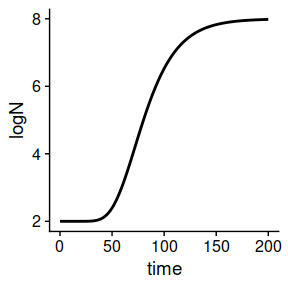The function uses gpplot, so it can be edited using layers as usual in the ggplot2 package. For instance, plot(static_prediction) + xlab("Storage time (h)") + ylab("Microbial count (log CFU/ml)") + theme_gray()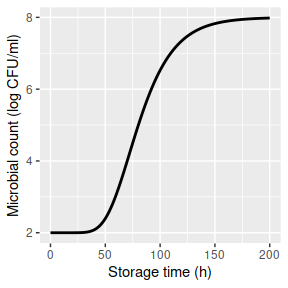The function includes additional arguments to edit the aesthetics of the plot. Please check the hepl page of plot.IsothermalGrowth for a full list of arguments. plot(static_prediction, line_col = "darkgreen", line_size = 2, line_type = 3) + xlab("Storage time (h)") + ylab("Microbial count (log CFU/ml)")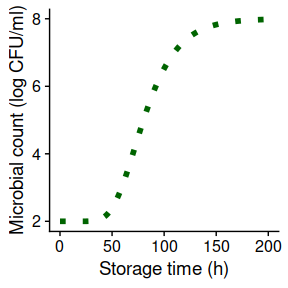Growth prediction under dynamic conditions The biogrowth package can also be used for simulating growth under dynamic environmental conditions using the predict_dynamic_growth() function. For this, this function combines primary and secondary growth models. It has 7 arguments: • times: Numeric vector of time points for the calculations. • env_conditions: A tibble describing the variation of the environmental conditions. • primary_pars: A named list describing the model parameters of the primary model. • secondary_models: A nested list defining the secondary model(s). • ...: Additional arguments passed to the numeric solver of the differential equation. • check: Whether to do some validity checks of model parameters (TRUE by default). • formula: A one-sided formula describing the x variable in env_conditions. The dynamic environmental conditions are defined using a tibble. It must have a defining the elapsed time and as many additional columns as needed for each environmental factor. By default, the column defining the time must be called time, although this can be changed using the formula argument. For the simulations, the value of the environmental conditions at time points not included in env_conditions is calculated by linear interpolation. In our simulation we will consider two environmental factors: temperature and pH. We can define their variation using this tibble. To illustrate the use of the formula argument, we will use Time for the column describing the elapsed time. my_conditions <- tibble(Time = c(0, 5, 40), temperature = c(20, 30, 35), pH = c(7, 6.5, 5) ) Then, the simulations would consider this temperature profile ggplot(my_conditions) + geom_line(aes(x = Time, y = temperature))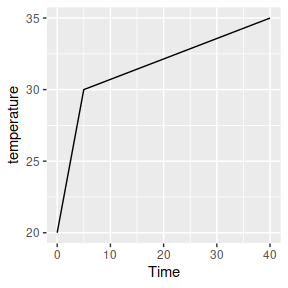And this pH profile ggplot(my_conditions) + geom_line(aes(x = Time, y = pH))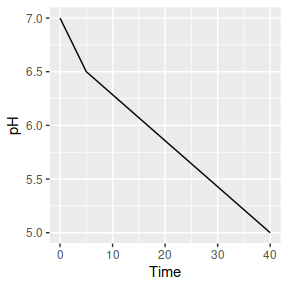We could define smoother profiles using additional rows. For time points outside of the range defined in env_conditions, the value at the closes extreme is used (rule=2 from approx function). For dynamic conditions, biogrowth uses the Baranyi growth model as primary model. This model requires the definition of two model parameters: the specific growth rate at optimum conditions (mu_opt) and the maximum population size (Nmax). Moreover, the initial values of the population size (N0) and the theoretical substance $$Q$$ (Q0) must be defined. Note that $$Q_0$$ is related to the duration of the lag phase under isothermal conditions by the identity $$\lambda = \ln \left( 1 + 1/q_0 \right)/\mu_{max}$$. For the predict_dynamic_growth()function, they all must be defined in a single list: my_primary <- list(mu_opt = .9, Nmax = 1e8, N0 = 1e0, Q0 = 1e-3) The next step is the definition of the secondary models. As already described above, biogrowth describes the variation of $$\mu$$ with temperature based on the gamma concept. Therefore, we need to define one secondary model per environmental condition. This must be done using a list. We define a list per environmental condition that defines the type of gamma model as well as the model parameters. The function secondary_model_data() can aid in the definition of the secondary models. For instance, we will define a gamma-type model for temperature as defined by Zwietering et al. (1992). This is done by including an item called model in the list and assigning it the value "Zwietering". Then, we define the values of the model parameters. In this case, we need the minimum (xmin) and optimum (xopt) cardinal values, as well as the order of the model (n) (this information can be retrieved using secondary_model_data). We define them using individual entries in the list: sec_temperature <- list(model = "Zwietering", xmin = 25, xopt = 35, n = 1) Next, we will define a CPM model for the effect of pH. Note that the model selection is for illustration purposes, not based on any scientific knowledge. First of all, we need to set the item model to "CPM". Then, we need to define the model parameters (note this model also needs xmax). sec_pH <- list(model = "CPM", xmin = 5.5, xopt = 6.5, xmax = 7.5, n = 2) The final step for the definition of the gamma-type secondary model is gathering all the individual models together in a single list and assigning them to environmental factors. Each element on the list must be named using the same column names as in env_conditions. Before, we had used the column names temperature and pH. Thus my_secondary <- list( temperature = sec_temperature, pH = sec_pH ) The final argument is the time points where to make the calculations. We can use a numeric vector with 1000 points between 0 and 50 for this: my_times <- seq(0, 50, length = 1000) Once we have defined every argument, we can call the predict_dynamic_growth() function. Because we are using Time to define the elapsed time in env_conditions, we must also define the .~Time in the formula argument. dynamic_prediction <- predict_dynamic_growth(my_times, my_conditions, my_primary, my_secondary, formula = . ~ Time) This function returns a list of class DynamicGrowth with several items: • simulation: A tibble with the results of the simulation. • gammas: A tibble describing the variation of each gamma factor through the simulation. • env_conditions: Environmental conditions used for the simulations. • primary_pars: Primary model parameters used for the simulations. • sec_models: Secondary model parameters used for the simulations. The results of the simulation can be retrieved from the simulation item: dynamic_prediction$simulation
#> # A tibble: 1,000 x 4
#>      time     Q     N  logN
#>     <dbl> <dbl> <dbl> <dbl>
#>  1 0      0.001     1     0
#>  2 0.0501 0.001     1     0
#>  3 0.100  0.001     1     0
#>  4 0.150  0.001     1     0
#>  5 0.200  0.001     1     0
#>  6 0.250  0.001     1     0
#>  7 0.300  0.001     1     0
#>  8 0.350  0.001     1     0
#>  9 0.400  0.001     1     0
#> 10 0.450  0.001     1     0
#> # … with 990 more rows

We can also visualize the simulation using the S3 method for plot:

plot(dynamic_prediction)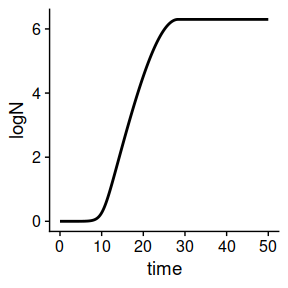The argument add_factor of the plot method can be used to plot the variation of a single environmental factor through storage. For that, one has to pass the name of the desired factor to the function. Note that this name must be identical to the one used for the columns in env_conditions. For instance, we can add the plot of temperature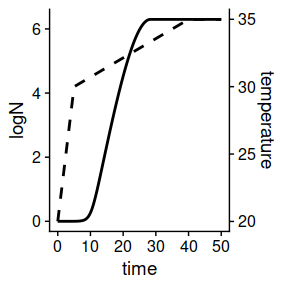The function includes several arguments to edit the aesthetics of the plot. A list of every argument can be found in the help page of plot.DynamicGrowth. The function returns a ggplot object, so it can be further edited using layers.

plot(dynamic_prediction,
ylims= c(0, 7),
label_y1 = "Microbial count (log CFU/ml)",
label_y2 = "Storage temperature (ºC)",
line_col = "lightgreen",
line_size = 2, line_type2 = 1
) +
xlab("Storage time (h)")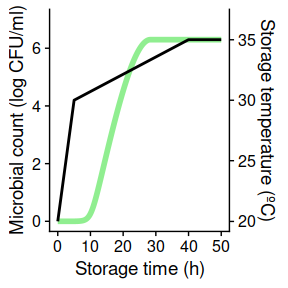Time to reach a given population size

It is usually of interest to calculate the time required for the population to reach a given size. The biogrowth package includes the function time_to_logcount() for this purpose. This function has 2 arguments:

• model: A model returned by predict_dynamic_growth() or predict_isothermal_growth().
• log_count: target population size.

For instance, we can use this function to estimate the time required to reach a population size of 2.5 log CFU/ml for the static prediction we did earlier:

time_to_logcount(static_prediction, 2.5)
#>  51.98105

Or the time required to reach 5 log CFU/ml in the dynamic prediction:

time_to_logcount(dynamic_prediction, 5)
#>  21.33296

If the value of log_count was outside the range of the simulations, time_to_logcount returns NA:

time_to_logcount(dynamic_prediction, 10)
#>  NA

Note that the calculations are based on linear interpolation of the simulated growth curve. Therefore, its accuracy is strongly dependent on the number of time points used for the simulation. It is recommended to plot the growth curve before doing this calculation. If the curve is not smooth in the area close to the target population size, the simulation should be repeated increasing the number of time points.

Fitting of primary models

The function fit_isothermal_growth() can be used to estimate the parameters of primary growth models from data obtained under static conditions. This function has 7 arguments:

• fit_data defines the data used for model fitting.
• model_name defines the primary model to use (according to primary_model_data()).
• starting_point defines the initial values of the model parameters (according to primary_model_data()).
• known_pars defines parameters that are considered known and are not fitted to the data.
• ... can be used to pass additional arguments to the modFit() function from the FME package (e.g. lower and upper bounds for the model parameters).
• check states whether to make some validity checks of the model parameters.
• formula defines the names of the x and y variables of the primary model.

The data used for model fitting is defined using the fit_data argument. It must be a tibble with one column defining the elapsed time (time by default), and another one defining the decimal logarithm of the population size (logN by default). For instance, we can use the following tibble, where the elapsed time uses the default column name (time) and the logarithm of the population size uses the name log_size.

my_data <- tibble(time = c(0, 25, 50, 75, 100),
log_size = c(2, 2.5, 7, 8, 8))

In case non-default names are used, they must be defined using the formula argument. The left handside of the equation defines the y-variable, and the right handside the x-variable of the primary model. For the tibble my_data, it would be defined as

my_formula <- log_size ~ time

The primary model is defined using model_name. For instance, we will use the Baranyi model in this example:

my_model <- "Baranyi"

The fit_isothermal_growth() function uses non-linear regression (through the modFit() function of the FME package), so it requires initial values for every model parameter to fit. In the case of the Baranyi model, the model parameters are: logN0, mu, lambda and logNmax (retrieved using primary_model_data("Baranyi")). The fit_isothermal_growth() function enables to fix any model parameter before model fit using the known_pars argument. This can be of interest, as growth models usually have issues related to parameter identifiability. For instance, we can fix the specific growth rate to 0.2 (no particular reason for this, just as a demonstration)

known <- c(mu = .2)

And fit the remaining model parameters

start = c(logNmax = 8, lambda = 25, logN0 = 2)

Once every model parameter has been defined, we can call the fit_isothermal_growth() function. The fitting is done based on the residuals of the logarithm of the population size.

static_fit <- fit_isothermal_growth(my_data, my_model,
start, known,
formula = my_formula
)

This function returns a list of class FitIsoGrowth with several items:

• data: data used for model fitting
• model: name of the primary model
• starting_point: starting point used for model fitting
• known: fixed model parameters
• fit: object returned by modFit()
• best_prediction: an instance of IsothermalGrowth corresponding to the fitted model.

The FitIsoGrowth class includes several S3 methods to help analyzing the results. The statistical information of the fit can be retrieved using summary()

summary(static_fit)
#>
#> Parameters:
#>         Estimate Std. Error t value Pr(>|t|)
#> logNmax  7.99600    0.06980  114.56 7.62e-05 ***
#> lambda  24.64154    0.74220   33.20 0.000906 ***
#> logN0    2.05084    0.09201   22.29 0.002007 **
#> ---
#> Signif. codes:  0 '***' 0.001 '**' 0.01 '*' 0.05 '.' 0.1 ' ' 1
#>
#> Residual standard error: 0.09879 on 2 degrees of freedom
#>
#> Parameter correlation:
#>         logNmax  lambda   logN0
#> logNmax 1.00000 0.05788 0.01589
#> lambda  0.05788 1.00000 0.76437
#> logN0   0.01589 0.76437 1.00000

Besides summary, it also includes methods for residuals, coef, vcov, deviance, fitted and predict to analize the model.

It also includes a plot() method to visually compare the data and the fitted curve

plot(static_fit)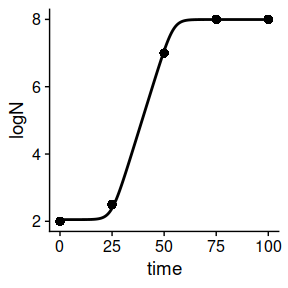The method accepts additional arguments to edit the aesthetics of the plot. A complete list of arguments can be found in the help page of plot. This plot can be edited passing additional arguments toplot.FitIsoGrowth.

plot(static_fit,
line_size = 2, point_col = "lightblue", point_size = 5)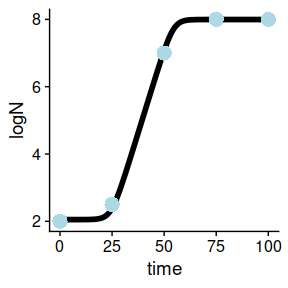Note this method returns an object of class ggplot. Hence, it can be edited using additional layers.

Fitting of secondary (gamma) models

The function fit_secondary_growth() can be used to estimate the parameters of secondary models defined using the gamma-approach based on a series of growth rates observed under various growth experiments under static conditions. This function has 8 arguments:

• fit_data: data used for the fit.
• starting_point: initial value of the model parameters
• known_pars: model parameters fixed (not fitted to the data).
• sec_model_names: type of secondary model for each environmental factor.
• transformation: transformation of the growth rate for the fit (square root by default).
• ...: additional arguments passed to modFit().
• check: whether to do some validity checks of the model parameters (TRUE by default).
• formula: a formula defining the column name defining the growth rate.

The fit_data argument must be a tibble containing the growth rates observed in several experiment under static environmental conditions. It must have one column describing the observed growth rate. Then, it must have as many additional columns as environmental factors included in the experiment. By default, the column describing the growth rate must be named mu. This can be changed using the formula argument, which is a one-sided formula, where the left handside defines the column name.

The biogrowth package includes the dataset example_cardinal to illustrate the data used by this function. It represents the specific growth rate (in log10 CFU/h) observed in several growth experiments under static environmental conditions, where each row represent one experiment. In this simulated dataset, two environmental factors were considered: temperature and pH.

data("example_cardinal")
#>   temperature pH           mu
#> 1    0.000000  5 9.768505e-04
#> 2    5.714286  5 2.624919e-03
#> 3   11.428571  5 0.000000e+00
#> 4   17.142857  5 1.530706e-04
#> 5   22.857143  5 2.301817e-05
#> 6   28.571429  5 3.895598e-04

In the example dataset, the series of experiments considered two environmental conditions: temperature and pH. Nonetheless, the fit_secondary_growth() function is entirely flexible with respect to the number of factors and their names. The only restriction is that the definition of the columns of the dataset and the secondary models is consistent.

The type of secondary model to use for each environmental factor is defined in the sec_model_names argument. It is a named vector whose names are the environmental factors and whose values define the model to use. The list of available models can be retrieved using secondary_model_data(). For this example, we will use a CPM for pH and an Zwietering model for temperature (this decision is not based on any scientific argument, just as demonstration of the functions in the package). Note that the names of the vector are identical to the column names of fit_data.

sec_model_names <- c(temperature = "Zwietering",
pH = "CPM")

The fit_secondary_growth() function estimates the values of the cardinal parameters, as well as the growth rate under optimal conditions using the modFit function from FME. As already mentioned, growth models usually have parameter identifiability issues. For that reason, the function enables fixing any model parameter to an arbitrary value before fitting the model. The model parameters to fit are defined using the known_pars argument. The remaining parameters, because of the use of non-linear regression for parameter estimation, require the definition of initial parameter values.

The growth rate under optimal conditions is named mu_opt. The remaining cardinal parameters are named according to the convention environ_factor+_+parameter(lower case). For instance, the minimum temperature for growth is temperature_xmin and the order of the CPM for pH is pH_n. Note that the environmental factor must be identical to the one used in sec_model_names.

For this example, we will consider that the growth rate under optimum conditions is known, as well as most of the the cardinal parameters for pH. Regarding temperature, we will only fix the order of the model.

known_pars <- list(mu_opt = 1.2,
temperature_n = 1,
pH_n = 2, pH_xmax = 6.8, pH_xmin = 5.2
)

The remaining model parameters will be fitted to reasonable initial values based on the range of the data.

my_start <- list(temperature_xmin = 5, temperature_xopt = 35,
pH_xopt = 6.5)

Finally, the transformation argument defines the transformation of the growth rate to use for model fitting. By default, the function applies a square root transformation, which has proved to stabilize the variance of microbial growth. Once the arguments have been defined, we can call the fit_secondary_growth() function. Note that, because we are using the default value of transformation, we do not need to define this argument. The same applies to formula, as the growth rate is named mu in example_cardinal.

fit_cardinal <- fit_secondary_growth(example_cardinal, my_start, known_pars, sec_model_names)

Before doing the calculations, this function does several validity checks of the model parameters, raising warnings or errors if there is some discrepancy between the parameter definition and the model requirements. If the fitting was successful, it returns an instance of FitSecondaryGrowth with 5 items:

• fit_results the object returned by modFit.
• secondary_model parameters of the secondary model.
• mu_opt_fit estimated growth rate under optimal storage conditions.
• data data used for the fit.
• transformation transformation applied to the growth rate before fitting.

This class incorporates several S3 method to ease visualization of results. The function summary() returns the statistical information of the fit.

summary(fit_cardinal)
#>
#> Parameters:
#>                  Estimate Std. Error t value Pr(>|t|)
#> temperature_xmin  5.31768    0.14965   35.53   <2e-16 ***
#> temperature_xopt 34.29640    0.76253   44.98   <2e-16 ***
#> pH_xopt           6.51109    0.01171  555.90   <2e-16 ***
#> ---
#> Signif. codes:  0 '***' 0.001 '**' 0.01 '*' 0.05 '.' 0.1 ' ' 1
#>
#> Residual standard error: 0.04026 on 61 degrees of freedom
#>
#> Parameter correlation:
#>                  temperature_xmin temperature_xopt    pH_xopt
#> temperature_xmin        1.000e+00          -0.1911 -2.376e-09
#> temperature_xopt       -1.911e-01           1.0000 -2.173e-01
#> pH_xopt                -2.376e-09          -0.2173  1.000e+00

Besides summary, it also includes methods for residuals, coef, vcov, deviance, fitted and predict.

The package includes S3 methods to plot the results. By default, a plot comparing observed and predicted values is shown

plot(fit_cardinal)
#> geom_smooth() using formula 'y ~ x'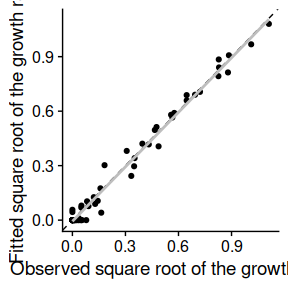In this plot, the dashed line is the line with intercept 0 and slope 1 where every point should fall in case of a perfect fit. The solid gray line is the regression line of predictions vs observations.

Alternatively, by passing the argument which=2, one can plot the observed and predicted counts as a function of the environmental factors

plot(fit_cardinal, which = 2)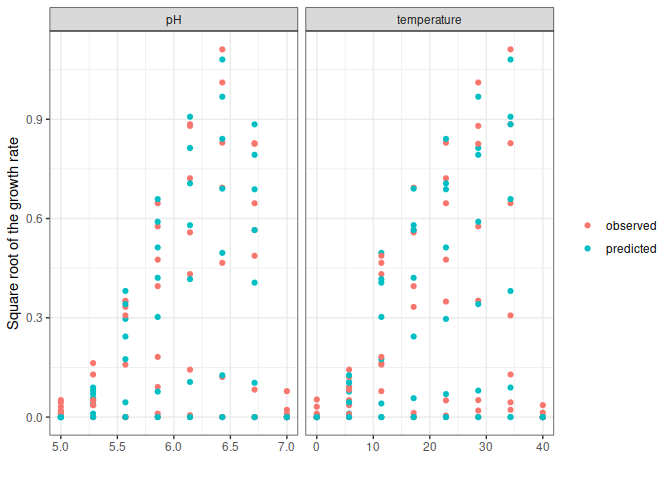A trend line can be added to this plot using the add_trend=TRUE argument:

plot(fit_cardinal, which = 2, add_trend = TRUE)
#> geom_smooth() using method = 'loess' and formula 'y ~ x'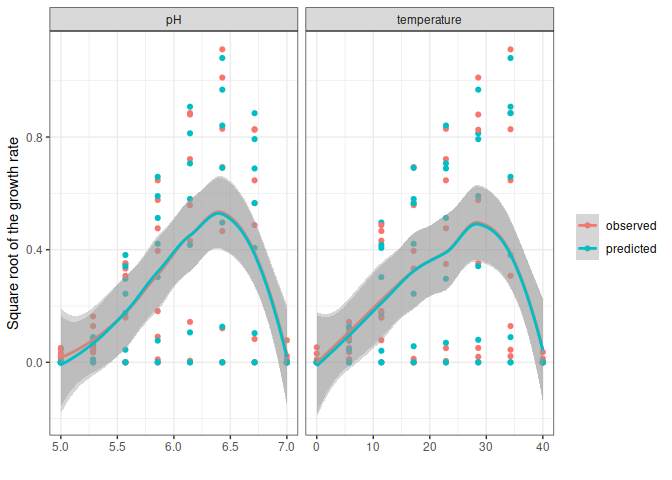Note that this line is not the predictions of the gamma model but a trend line based on the observations or the predictions. Therefore, it can be used to compare the tendency of the model predictions against the one of the observations, but it should not be used for model predictions (the predict method should be used instead).

One-step fitting under dynamic conditions

The function fit_dynamic_growth() can be used to estimate the parameters of both the primary and the secondary model based on a growth experiment obtained under dynamic conditions. This function has 8 arguments:

• fit_data: data used for model fitting.
• env_conditions: variation of the environmental conditions during the experiment.
• starting_point: initial value of the model parameters.
• known_pars: known model parameters (i.e. not fitted).
• sec_model_names: type of secondary model for each environmental factor.
• ...: additional arguments passed to modFit.
• check: whether to do some validity checks of the model parameters (TRUE by default).
• formula: a formula defining the x and y variables of the primary model.

The arguments env_conditions and fit_data are tibbles that describe, respectively, the experimental design and the observations. The biogrowth package includes two example datasets to illustrate the input requirements for this function.

data("example_dynamic_growth")
data("example_env_conditions")

The tibble passed to the argument env_conditions must have a column defining the elapsed time (time by default) and as many additional columns as environmental factors. The fit_dynamic_growth() function is totally flexible with respect to the number of factors or the way they are named. The only requirement is that the definition of every argument is consistent. In the case of example_env_conditions, this dataset considers two factors: temperature and water activity (aw).

#> # A tibble: 3 x 3
#>    time temperature    aw
#>   <dbl>       <dbl> <dbl>
#> 1     0          20  0.99
#> 2     5          30  0.95
#> 3    15          35  0.9

Note that for the calculations this function joins the data points by linear interpolation, as shown in this plot:

ggplot(example_env_conditions, aes(x = time, y = temperature)) +
geom_line() +
geom_point()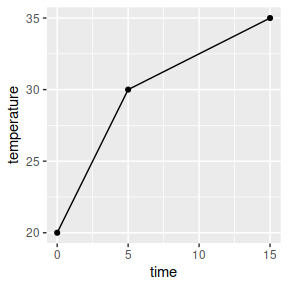The tibble passed to the argument fit_data must have one column defining the elapsed time (time by default) and one defining the logarithm of the population size (logN by default). Different column names can be defined using the formula argument. For instance, formula=log_size ~ Time would mean that the elapsed time is called “Time” and the logarithm of the population size is called “log_size”. Note that the name of the column describing the elapsed time in fit_data must be identical to the one in env_conditions.

#> # A tibble: 6 x 2
#>    time     logN
#>   <dbl>    <dbl>
#> 1 0      0.0670
#> 2 0.517 -0.00463
#> 3 1.03  -0.0980
#> 4 1.55  -0.0986
#> 5 2.07   0.111
#> 6 2.59  -0.0465

The type of secondary model for each environmental factor is defined by the argument sec_model_names. This argument is a named vector where the names refer to the environmental factor and the value to the type of model. Supported models can be retrieved using secondary_model_data(). In this example we will use cardinal models for both environmental factors. Note that the names of this vector must be identical to the columns in env_conditions.

sec_model_names <- c(temperature = "CPM",
aw= "CPM")

As already mentioned, growth models usually have to deal with parameter identifiability issues. For that reason, the fit_dynamic_growth() function enables to fit or fix any model parameter. This distinction is made using the arguments known_pars (fixed parameters) and starting_point (fitted parameters). Note that every parameter of the primary and secondary model must be in either of these arguments without duplication.

This function uses the Baranyi primary model. It has two variables that need initial values (N0 and Q0) and one primary model parameter (Nmax). The specific growth rate is described using the gamma concept. This requires the definition of its value under optimal conditions (mu_opt) as well as the cardinal parameters for each environmental factor. They must be defined as factor+_+parameter name. For instance, the minimum water activity for growth must be written as aw_xmin.

In this example we will consider the model parameters of the primary model as known. For the secondary model for water activity, we will only consider the optimum value for growth as unknown. Finally, for the effect of temperature, we will consider the order and xmax are known:

known_pars <- list(Nmax = 1e4,  # Nmax for primary model
N0 = 1e0, Q0 = 1e-3,  # Initial values of the primary model
mu_opt = 4, # mu_opt of the gamma model
temperature_n = 1, temperature_xmax = 40,  # Secondary model for temperature
aw_xmax = 1, aw_xmin = .9, aw_n = 1  # Secondary model for water activity
)

Then, the remaining model parameters must be defined in starting_points. Due to the use of non-linear regression for model fitting, it is required to define initial values for these parameters. They can be defined based on previous experience or preliminary numerical simulations.

my_start <- list(temperature_xmin = 25, temperature_xopt = 35,
aw_xopt = .95)

Once every model parameter has been defined, we can call the fit_dynamic_growth() function.

my_dyna_fit <- fit_dynamic_growth(example_dynamic_growth, example_env_conditions,
my_start,
known_pars,
sec_model_names)

The function does some checks of the validity of the model parameters (can be turned off using check=FALSE), raising errors if the model definition does not follow the requirements of the functions. If the fitting was successful, it returns an instance of FitDynamicGrowth with 7 items:

• fit_results: object returned by modFit().
• best_prediction: an instance of DynamicGrowth with the prediction corresponding to the fitted model.
• data: data used to fit the model.
• env_conditions: data used to describe the environmental conditions.
• starting: starting values used for parameter estimation.
• known: model parameters considered known.
• sec_models: type of secondary model for each environmental factor.

FitDynamicGrowth includes an S3 method for summary that returns the statistical information of the fit.

summary(my_dyna_fit)
#>
#> Parameters:
#>                  Estimate Std. Error t value Pr(>|t|)
#> temperature_xmin 26.43224    3.46652   7.625 3.35e-08 ***
#> temperature_xopt 33.85365   10.89999   3.106  0.00443 **
#> aw_xopt           0.99147    0.05574  17.786  < 2e-16 ***
#> ---
#> Signif. codes:  0 '***' 0.001 '**' 0.01 '*' 0.05 '.' 0.1 ' ' 1
#>
#> Residual standard error: 0.1478 on 27 degrees of freedom
#>
#> Parameter correlation:
#>                  temperature_xmin temperature_xopt aw_xopt
#> temperature_xmin           1.0000          -0.9897  0.9762
#> temperature_xopt          -0.9897           1.0000 -0.9971
#> aw_xopt                    0.9762          -0.9971  1.0000

Besides summary, it also includes methods for residuals, coef, vcov, deviance, fitted and predict to analyze the fitted model. Moreover, it includes a plo method to compare the model predictions against the data used for the fit:

plot(my_dyna_fit)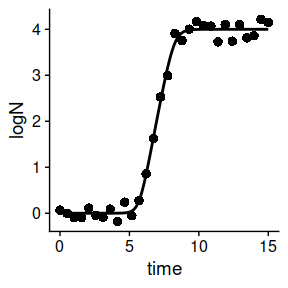The variation of the environmental factor can be plotted alongside the previous plot. For that, the name of the environmental factor must be passed to add_factor. Note that the value passed must be identical to the one defined in the previous arguments. The function provides additional arguments to edit the aesthetics of the plot (a full list can be retrieved from the help page of plot.FitDynamicGrowth). The function returns an instance of ggplot, so it can be edited using additional layers.

label_y1 = "Log count (log CFU/ml)",
label_y2 = "Water activity",
line_col = "pink",
line_col2 = "yellow",
point_col = "lightgreen") +
theme_dark()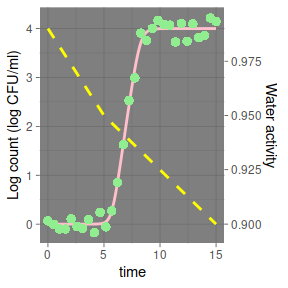Global fitting of various (dynamic) experiments

The function fit_multiple_growth() can be used to fit one growth model to data gathered in various experiments performed under dynamic conditions. It has several arguments:

• starting_point starting values of the model parameters to fit.
• experiment_data a nested list describing the experimental data and environmental conditions.
• known_pars vector of parameters that are fixed (i.e. not fitted).
• sec_model_names definition of the secondary models for each condition.
• ... additional arguments passed to FME::modFit.
• check whether to do validity checks of the model parameters (TRUE by default).
• formula defines the x and y variables of the primary model.

The data to use for the fit is described using the experiment_data argument. It is a nested list with as many elements as experiments. The dataset multiple_experiments serves as a convenient example for this function.

data("multiple_experiments")

Each experiment is described using a list with two elements: data and conditions. The element data describe the observed variation in the population size using the same convention as fit_data in fit_dynamic_growth().

ggplot(multiple_experiments[]$data) + geom_point(aes(x = time, y = logN))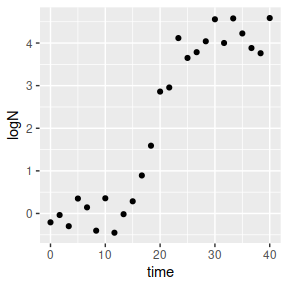The element conditions describes the (dynamic) environmental conditions during storage. It follows the same requirements as env_conditions in fit_dynamic_growth(). Although the function is flexible regarding the number of environmental factors or the column names, they must be consistent for every element in the list. As already mentioned, for every simulation, the values of environmental conditions at times not included in the data frame are calculated by linear interpolation, as illustrated in the next plot. multiple_experiments[]$conditions %>%
pivot_longer(-time, names_to = "condition", values_to = "value") %>%
ggplot() +
geom_line(aes(x = time, y = value)) +
facet_wrap("condition", scales = "free")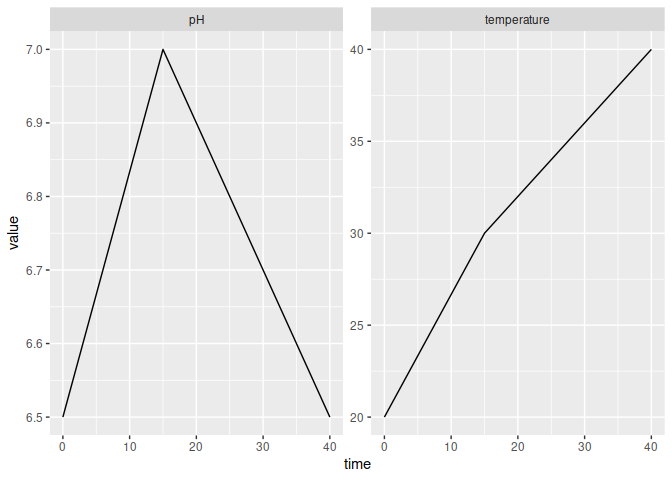The secondary models are defined using sec_model_names, following the same convention as in predict_dynamic_growth(). This argument is a named vector whose names are identical to those in the experimental data and whose values corresponds to valid identifiers according to seccondary_model_data(). For this example, we will use a CPM model for both pH and temperature (for no particular scientific reason).

sec_names <- c(temperature = "CPM", pH = "CPM")

The next step is the definition of model parameters. This is done using the starting_point (for parameters to estimate) and known_pars (for known parameters) arguments, which are lists. Every parameter of both the primary and secondary models must be included in either of these arguments. The format for parameter definition is identical to the one of fit_dynamic_inactivation.

For this example, we will only fit the maximum specific growth rate and the optimum temperature for growth (for no particular scientific reason).

known <- list(Nmax = 1e8, N0 = 1e0, Q0 = 1e-3,
temperature_n = 2, temperature_xmin = 20, temperature_xmax = 35,
pH_n = 2, pH_xmin = 5.5, pH_xmax = 7.5, pH_xopt = 6.5)

start <- list(mu_opt = .8, temperature_xopt = 30)

Once every argument has been defined, we can call the fit_multiple_growth() function. To aid convergence, we will define upper and lower limits for the parameter estimates (see the help page of modFit).

global_fit <- fit_multiple_growth(start, multiple_experiments, known, sec_names,
lower = c(.5, 25),
upper = c(1, 33))

This function does several validity checks of the model parameters (can be turned off passing check=FALSE), raising errors if there is any mismatch between the model definition and the requirements of the functions. If the fit was successful, it returns an instance of FitMultipleDynamicGrowth with several elements.

• fit_results: object returned by modFit.
• best_prediction: instance of DynamicGrowth with the prediction of the fitted model.
• data: data used for model fitting.
• starting: starting guesses of the model parameters.
• known: fixed model parameters.
• sec_models: names of the secondary models for each factor.

It includes several S3 methods for visualization and statistical analysis. The statistical information can be accessed using summary

summary(global_fit)
#>
#> Parameters:
#>                  Estimate Std. Error t value Pr(>|t|)
#> mu_opt            0.54196    0.01222   44.35   <2e-16 ***
#> temperature_xopt 30.62395    0.18727  163.53   <2e-16 ***
#> ---
#> Signif. codes:  0 '***' 0.001 '**' 0.01 '*' 0.05 '.' 0.1 ' ' 1
#>
#> Residual standard error: 0.4282 on 48 degrees of freedom
#>
#> Parameter correlation:
#>                  mu_opt temperature_xopt
#> mu_opt           1.0000           0.8837
#> temperature_xopt 0.8837           1.0000

Besides summary, it includes methods for residuals, coef, vcov, deviance, fitted and predict.

Moreover, the predictions of the fitted model can be compared against the data using plot.

plot(global_fit)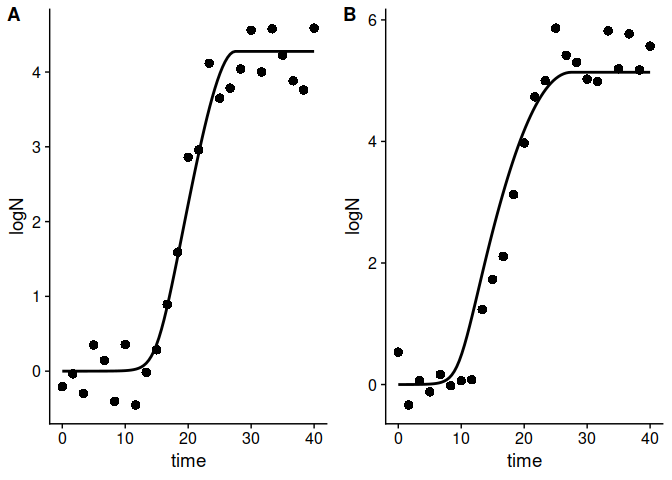This function generates an individual plot for each experiment. Any environmental factor can be included in the plot by passing the name of the factor to the add_factor argument. Note that the name must be identical to the one used for model definition.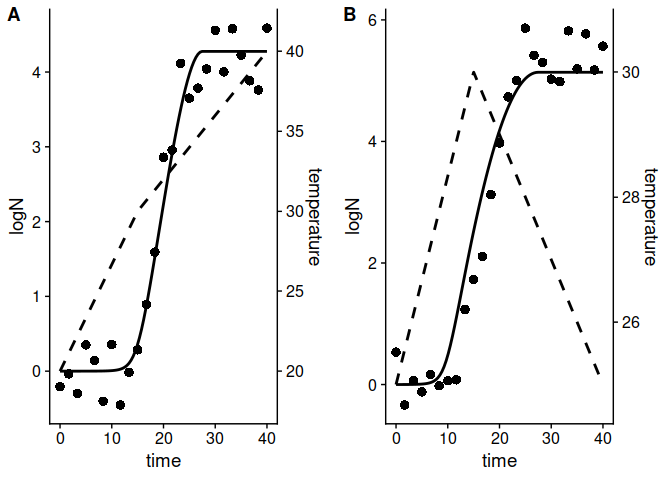The function includes additional arguments to edit several aesthetics of the plot. A full list of arguments can be found in the help page of plot.FitDynamicGrowth. The function returns an object of class ggplot, so it can be edited further using additional layers.

label_x = "Storage time (h)",
label_y1 = "Size of the population (log CFU/g)",
label_y2 = "Temperature (ºC)",
line_col = "maroon", line_size = 2,
line_type2 = 1, line_col2 = "darkgrey"
)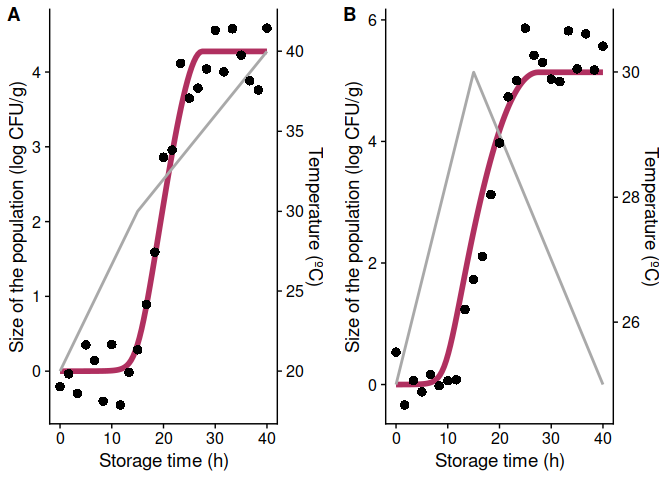Stochastic modelling

Stochastic prediction under static conditions

Due to the relevance of uncertainty and variability, Quantitative Microbial Risk Assessment usually requires the calculation of stochastic simulations. The function predict_stochastic_growth enables to include parameter uncertainty on the model predictions. It has 6 arguments:

• model_name defines the primary growth model,
• times defines the time points where to make the calculations,
• n_sims defines the number of Monte Carlo simulations,
• pars defines the distribution of the model parameters,
• corr_matrix correlation matrix between the model parameters. By default, this argument is set to an identity matrix (i.e. no correlation between parameters).
• check states whether to do validity checks of the model parameters (TRUE by default).

The calculations are done by taking a sample of size n_sims of the model parameters according to a multi-variate normal distribution. For simulation, the microbial growth is predicted and the quantiles of the predicted population size is used as an estimate of the credible interval.

For this example, we will use the modified Gompertz model

my_model <- "modGompertz"

The pars argument defines the distribution of the model parameters. It must be a tibble with 4 columns (par, mean, sd and scale) and as many rows as model parameters. Then, for the modified Gompertz model, we will need 4 rows. The column par defines the parameter that is defined on each row. It must be a parameter identifier according to primary_model_data(). This function considers that each model parameter follows a marginal normal distribution with the mean defined in the mean column and the standard deviation defined in sd. This distribution can be defined in log-scale (by setting the value in scale to “log”), square-root scale (“sqrt”) or in the original scale (“original”). Note that, in order to omit the variability/uncertainty of any model parameter, one just has to set its corresponding standard error to zero.

pars <- tribble(
~par, ~mean, ~sd, ~scale,
"logN0", 0, .2, "original",
"mu", 2, .3, "sqrt",
"lambda", .5, .1, "log",
"C", 6, .5, "original"
)

For the time points, we will take 100 points uniformly distributed between 0 and 200:

my_times <- seq(0, 15, length = 100)

For the example, we will set the number of simulations to 1000. Nevertheless, it is advisable to repeat the calculations for various number of simulations to ensure convergence.

n_sims <- 1000

Once the arguments have been defined, we can call the predict_stochastic_growth() function.

stoc_growth <- predict_stochastic_growth(my_model, my_times, n_sims, pars)

Before doing any calculations, predict_stochastic_growth() makes several validity checks of the model parameters (this can be turned off by passing check=FALSE). It returns an instance of StochasticGrowth with several items:

• sample: sample of model parameters used for the simulations
• simulations: results of the individual simulations
• quantiles: quantiles of the population size predicted in the simulations
• model: model used for the simulations
• mus: expected values of the model parameters used for the simulations.
• sigma: variance-covariance matrix used for the simulations

This class implements an S3 method for plot that can be used to visualize the credible intervals

plot(stoc_growth)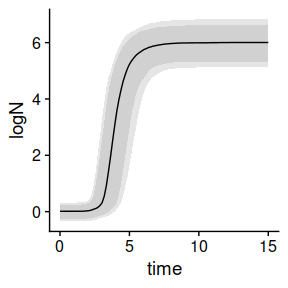In this plot, the solid line represents the mean of the simulations. Then, the two shaded areas represent, respectively, the space between the 10th and 90th, and the 5th and 95th quantiles.

The plot method includes additional arguments to edit the aesthetics of the plot.

plot(stoc_growth, ribbon80_fill = "purple", ribbon90_fill = "pink", alpha80 = .8)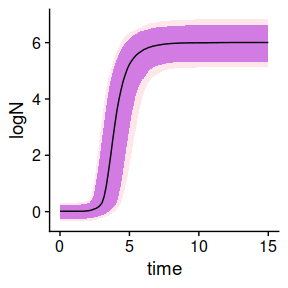By default, the function considers that there is no correlation between the model parameters. This can be varied by defining a correlation matrix. Note that the rows and columns of this matrix are defined in the the same order as in pars, and the correlation is defined in the scale of pars. For instance, we can define a correlation of -0.7 between the square root of $$\mu$$ and the logarithm of $$\lambda$$:

my_cor <- matrix(c(1,   0,    0, 0,
0,   1, -0.7, 0,
0, -0.7,   1, 0,
0,    0,   0, 1),
nrow = 4)

Then, we can include it in the call to the function

stoc_growth2 <- predict_stochastic_growth(my_model, my_times, n_sims, pars, my_cor)

plot(stoc_growth2)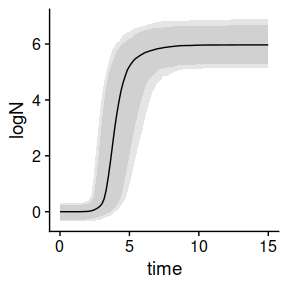One-step fitting using MCMC algorithms

Numerical algorithms based on Markov Chains have been suggested as an alternative to non-linear regression for dynamic models. For that reason, biogrowth includes the function fit_MCMC_growth() that uses the interface included in the FME package to the Adaptive Monte Carlo algorithm by Haario et al. (2006). The arguments and the requirements of this function are identical to those of fit_dynamic_growth(). The only difference is that this function has the additional argument niter, which defines the number of samples from the Markov Chain. Hence, we will repeat the previous code to define the model parameters and the data.

data("example_dynamic_growth")
data("example_env_conditions")

sec_model_names <- c(temperature = "CPM",
aw= "CPM")

known_pars <- list(Nmax = 1e4,  # Primary model
N0 = 1e0, Q0 = 1e-3,  # Initial values of the primary model
mu_opt = 4, # mu_opt of the gamma model
temperature_n = 1, temperature_xmax = 40, # Secondary model for temperature
aw_xmax = 1, aw_xmin = .9, aw_n = 1  # Secondary model for water activity
)

my_start <- list(temperature_xmin = 25,
temperature_xopt = 35,
aw_xopt = .95)

Then, we can call the fit_MCMC_growth() using these arguments plus the argument niter that we will set to 100.

my_MCMC_fit <- fit_MCMC_growth(example_dynamic_growth, example_env_conditions,
my_start,
known_pars,
sec_model_names,
niter = 100)
#> number of accepted runs: 11 out of 100 (11%)

This function returns an instance of FitDynamicGrowthMCMC with 7 entries:

• fit_results: object returned by modMCMC().
• best_prediction: an instance of DynamicGrowth with the prediction corresponding to the fitted model.
• env_conditions: a tibble with the environmental conditions used for the simulations.
• data: data used to fit the model.
• starting: starting values used for parameter estimation.
• known: model parameters considered known.
• sec_models: type of secondary model for each environmental factor.

This class implements several S3 methods to aid in the the visualization of the results. A call to summary() returns the statistics of the Markov Chain.

summary(my_MCMC_fit)
#>      temperature_xmin temperature_xopt    aw_xopt
#> mean        27.579847        27.818451 0.95876831
#> sd           1.123397         2.623738 0.03852854
#> min         24.705271        24.042277 0.88510148
#> max         29.613602        35.275504 1.01202920
#> q025        27.752607        25.754117 0.90931392
#> q050        27.792468        25.754117 0.97325894
#> q075        27.792468        30.130946 0.97325894

Moreover, it includes methods for residuals, coef, vcov, deviance, fitted and predict. It also includes a plot method to compare the data against the fitted model.

plot(my_MCMC_fit)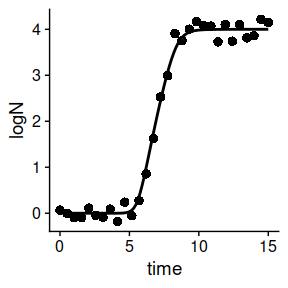As well as for fit_dynamic_growth(), the environmental conditions can be added to the plot using the add_factor argument. Other aesthetics can be edited passing additional arguments to plot (a full list can be found in the help page of plot.FitDynamicGrowthMCMC).

point_col = "steelblue", point_shape = 2, point_size = 6)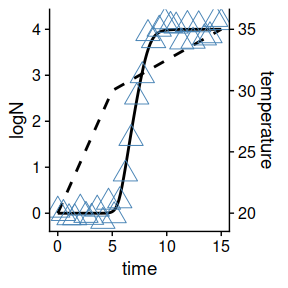Global fitting using MCMC algorithms

Following the same logic as with the fit_MCMC_growth() function, fit_multiple_growth_MCMC() serves as an alternative to fit_multiple_growth() that uses an MCMC fitting algorithm instead of non-linear regression. The arguments of this function are identical to those of fit_multiple_growth() with the addition of niter, which defines the number of iterations from the MCMC sample.

Therefore, the definition of the data to use for the fit is identical.

data("multiple_experiments")

As well as the model definition.

## For each environmental factor, we need to defined a model

sec_names <- c(temperature = "CPM", pH = "CPM")

## Any model parameter can be fixed

known <- list(Nmax = 1e8, N0 = 1e0, Q0 = 1e-3,
temperature_n = 2, temperature_xmin = 20, temperature_xmax = 35,
pH_n = 2, pH_xmin = 5.5, pH_xmax = 7.5, pH_xopt = 6.5)

## The rest require starting values for model fitting

start <- list(mu_opt = .8, temperature_xopt = 30)

Then, the function can be called. Note that the MCMC algorithm is stochastic, so we will set the seed before fitting to grant reproducibility. Additionally, we will define upper and lower bounds for this function by passing the arguments lower and upper to modMCMC. For further ways to edit the fitting, please check the help page of modMCMC().

set.seed(12412)
global_MCMC <- fit_multiple_growth_MCMC(start, multiple_experiments, known, sec_names,
niter = 100,
lower = c(.2, 29),  # lower limits of the model parameters
upper = c(1.6, 34))  # upper limits of the model parameters
#> number of accepted runs: 14 out of 100 (14%)

This function returns a list of class FitMultipleDynamicGrowthMCMC with the same entries as FitMultipleDynamicGrowth. It also implements S3 methods to inspect the parameter estimates

summary(global_MCMC)
#>          mu_opt temperature_xopt
#> mean 0.54824071       30.3830315
#> sd   0.08199758        0.7297392
#> min  0.45625004       29.0379803
#> max  0.80000000       33.1855454
#> q025 0.47736567       29.6356637
#> q050 0.51539399       30.0434791
#> q075 0.57479388       31.1219113

Or to plot the predictions of the fitted model against the data.

plot(global_MCMC)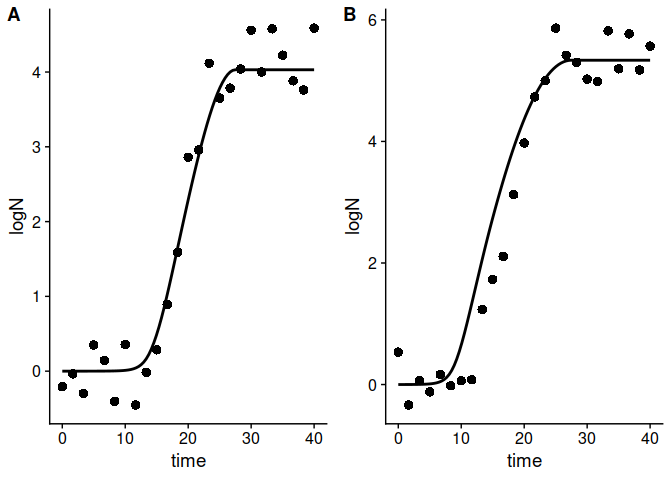Any environmental factor can be included in the plot using the add_factor argument. Also, the aesthetics of the plot can be edited passing additional arguments to plot (see the help page of plot.FitMultipleGrowthMCMC).

line_col = "grey",
line_col2 = "blue", line_size2 = .5, line_type2 = 3)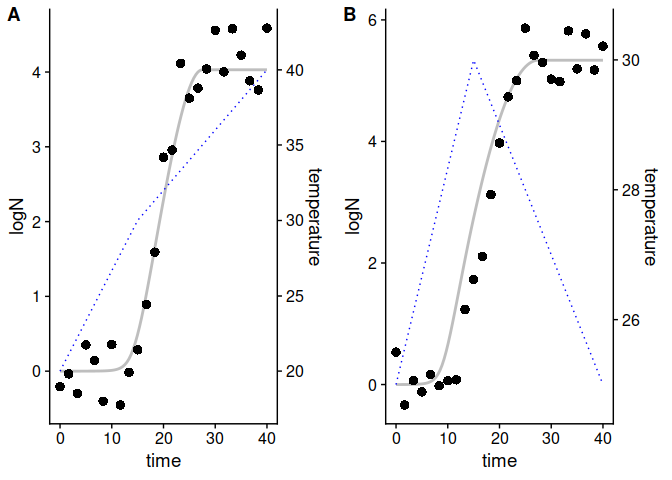Stochastic prediction based on an MCMC fit

The function predict_MCMC_growth() makes stochastic predictions based on parameter distributions estimated using fit_MCMC_growth() or fit_multiple_growth_MCMC(). This function has 5 arguments:

• MCMCfit an instance of FitDynamicGrowthMCMC returned by fit_MCMC_growth(), or an instance of FitMultipleGrowthMCMC returned by fit_multiple_growth_MCMC().
• times vector of time points for the calculations.
• env_conditions tibble describing the (dynamic) environmental conditions.
• niter number of samples for the Monte Carlo calculations.
• newpars can be used to use different parameter values to those used for model fitting.

For this first example, we will use the same data we used previously to illustrate the use of the fit_MCMC_growth() function. The environmental conditions were defined by example_env_conditions

example_env_conditions
#> # A tibble: 3 x 3
#>    time temperature    aw
#>   <dbl>       <dbl> <dbl>
#> 1     0          20  0.99
#> 2     5          30  0.95
#> 3    15          35  0.9

This function estimates the credible intervals based on the quantiles of the predicted population size at each time point. Hence, their precision depends on the number of time points and the number of simulations. If the number of time points is too low, the prediction interval would not be “smooth”. On the other hand, if the number of simulations is too low, the credible interval would vary between repetitions of the same calculation.

As an example, we will use 5 time points uniformly distributed between 0 and 15

my_times <- seq(0, 15, length = 5)

and 100 iterations.

niter <- 100

Once we have defined every argument, we can call the predict_MCMC_growth() function.

my_MCMC_prediction <- predict_MCMC_growth(my_MCMC_fit,
my_times,
example_env_conditions,
niter)

This function returns an instance of MCMCgrowth with 5 entries:

• sample a tibble with the sample of model parameters used for the simulations.
• simulations a tibble with the results of every individual simulation used.
• quantiles a tibble providing the calculated quantiles (5th, 10th, 50th, 90th, 95th) of the population size for each time point.
• model the instance of FitDynamicGrowthMCMC used for the predictions.
• env_conditions a tibble with the environmental conditions of the simulations.

Hence, the quantiles at each time point can be retrieved from quantiles

my_MCMC_prediction$quantiles #> # A tibble: 5 x 7 #> time q50 q10 q90 q05 q95 m_logN #> <dbl> <dbl> <dbl> <dbl> <dbl> <dbl> <dbl> #> 1 0 0 0 0 0 0 0 #> 2 3.75 0 0 0.00187 0 0.00187 0.000396 #> 3 7.5 2.78 2.54 3.60 2.54 3.99 2.85 #> 4 11.2 4.00 3.99 4.00 3.99 4.00 4.00 #> 5 15 4.00 4.00 4 4.00 4 4.00 This class implements an S3 method for plot to visualize the prediction interval. plot(my_MCMC_prediction)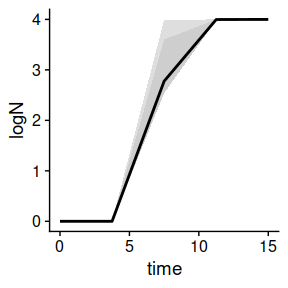In this plot, the solid line represents the median of the simulations, whereas the two shaded areas represent the space between the 10th and 90th, and 5th and 95th quantiles. As shown in this plot, the prediction interval is far from smooth. The reason for that is the low number of time points used for the calculations. Consequently, we will repeat them using 100 time points instead of 5: better_prediction <- predict_MCMC_growth(my_MCMC_fit, seq(0, 15, length = 100), example_env_conditions, niter) If we visualize the new prediction interval plot(better_prediction)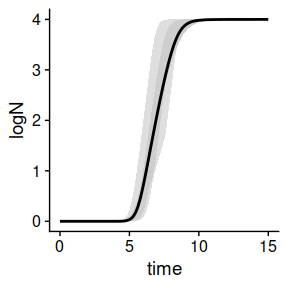it is now smoother. However, the prediction interval is still odd. Even if it is smooth, there are several inflection points that are hard to justify based on the model equations. They are the result of the low number of Monte Carlo samples used for the simulations. Hence, this number should be increased to obtain reliable intervals (not done in this vignette due to excessive compilation time). By default, the predict_MCMC_growth function uses every parameter value estimated from the fit. This can be a limitation for simulations. For instance, the initial population size of a population of pathogenic species in an experiment is much higher than the one usually found in a food product. Also, it could be of interest to disregard the uncertainty of one parameter estimate. For that reason, the function includes the newpars argument, which can be used to assign a value (disregarding uncertainty) to one or more parameters. For instance, we could define an initial population size of 10, and a $$aw_{opt}$$ of 0.96. other_prediction <- predict_MCMC_growth(my_MCMC_fit, seq(0, 15, length = 100), example_env_conditions, niter, newpars = list(aw_xopt = .96, N0 = 10 ) ) plot(other_prediction)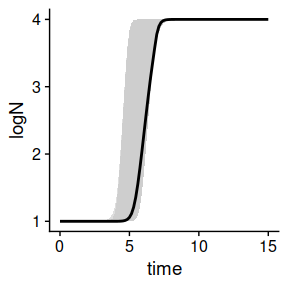Distributions of times to reach a certain count In many cases, the elapsed time required to reach a critical population size is usually of interest. For stochastic simulations, this time is not defined by a single value but by a probability distribution that can be estimated using the distribution_to_logcount() function. This function has two arguments: • model: an object returned by predict_stochastic_growth() or fit_MCMC_growth(). • log_count: target population size. For instance, we can use this function to get the distribution of times required to reach a population size of 4 log CFU/g based on the simulations we did before and saved in stoc_growth time_distrib <- distribution_to_logcount(stoc_growth, 3) This function returns an instance of TimeDistribution with 2 items: distribution includes a numeric vector with the distribution of times to reach log_count. summary includes summary statistics of distribution. Hence, we can observe the summary time_distrib$summary
#> # A tibble: 1 x 5
#>   m_time sd_time med_time   q10   q90
#>    <dbl>   <dbl>    <dbl> <dbl> <dbl>
#> 1   4.02   0.765     3.93  3.14  5.01

Alternatively, this class implements an S3 method to visualize the distribution of times:

plot(time_distrib)
#> stat_bin() using bins = 30. Pick better value with binwidth`.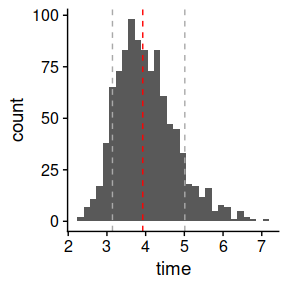In this plot, the vertical red line illustrates the median of the distribution and the grey lines the 10th and 90th quantile.

The width of the bins can be changed using the bin_width argument.

plot(time_distrib, bin_width = .5)